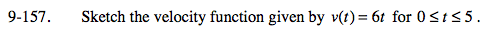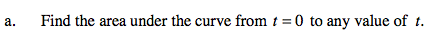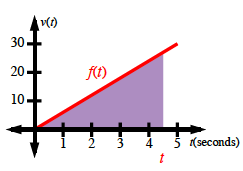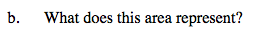### Home > PCT > Chapter Ch9 > Lesson 9.3.5 > Problem9-157

9-157.$\text{Area} = \frac{(\text{base})(\text{height})}{2}$

$\text{Area} = \frac{(t)(f(t))}{2}$

$\text{Area} = \frac{(t)(6t)}{2}$

Area = 3t2It represents distance traveled.